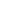NSNumber

 Inherits From: NSValue : NSObject Conforms To: NSCopying NSMutableCopying Declared In: foundation/NSValue.h

 Class Description

 NSNumber objects provide an object-oriented wrapper for C number data types. NSNumber, which inherits from NSValue, provides methods for creating number objects that contain data of a specified type. It also provides methods for extracting data from a number object and casting the data to be of a particular type. For comparing two number objects, NSNumber provides the compare: method that returns an ordered comparison result.

 Instance Variables

 None declared in this class.

 NSCopyingcopycopyWithZone:
 NSMutableCopyingmutableCopymutableCopyWithZone:

 Method Types

 Allocating and initializing + numberWithBool:
 + numberWithChar: + numberWithDouble: + numberWithFloat: + numberWithInt: + numberWithLong: + numberWithLongLong: + numberWithShort: + numberWithUnsignedChar: + numberWithUnsignedInt: + numberWithUnsignedLong: + numberWithUnsignedLongLong: + numberWithUnsignedShort:

 Accessing databoolValuecharValuedoubleValuefloatValueintValuelongLongValuelongValueshortValuestringValueunsignedCharValueunsignedIntValueunsignedLongLongValueunsignedLongValueunsignedShortValue

 Comparing datacompare:

 Class Methods

 numberWithBool:
 + numberWithBool:(BOOL)value

 Creates and returns a number object containing value of the type BOOL. numberWithChar:
 + numberWithChar:(char)value

 Creates and returns a number object containing value of the type char. This excerpt initializes a number object to contain the character `K'.NSNumber* numObj;char myChar = `K';numObj = [NSNumber numberWithChar:myChar];

 See also:numberWithUnsignedChar: numberWithDouble:
 + numberWithDouble:(double)value

 Creates and returns a number object containing value of the type double. numberWithFloat:
 + numberWithFloat:(float)value

 Creates and returns a number object containing value of the type float. numberWithInt:
 + numberWithInt:(int)value

 Creates and returns a number object containing value of the type int. See also:numberWithUnsignedInt: numberWithLong:
 + numberWithLong:(long)value

 Creates and returns a number object containing value of the type long. See also:numberWithUnsignedLong: numberWithLongLong:
 + numberWithLongLong:(long long)value

 Creates and returns a number object containing value of the type long long. See also:numberWithUnsignedLongLong: numberWithShort:
 + numberWithShort:(short)value

 Creates and returns a number object containing value of the type short. See also:numberWithUnsignedShort: numberWithUnsignedChar:
 + numberWithUnsignedChar:(unsigned char)value

 Creates and returns a number object containing value of the type unsigned char. See also:numberWithChar: numberWithUnsignedInt:
 + numberWithUnsignedInt:(int)value

 Creates and returns a number object containing value of the type unsigned int. See also:numberWithInt: numberWithUnsignedLong:
 + numberWithUnsignedLong:(unsigned long)value

 Creates and returns a number object containing value of the type unsigned long. See also:numberWithLong: numberWithUnsignedLongLong:
 + numberWithUnsignedLongLong:(unsigned long long)value

 Creates and returns a number object containing value of the type unsigned long long. See also:numberWithLongLong: numberWithUnsignedShort:
 + numberWithUnsignedShort:(unsigned short)value

 Creates and returns a number object containing value of the type unsigned short. See also:numberWithShort:

 Instance Methods

 boolValue(BOOL)boolValue

 Returns a BOOL value from a number object. charValue(char)charValue

 Returns a char value from a number object. See also:unsignedCharValue compare:(NSComparisonResult)compare:(NSNumber *)other

 Compares the receiver to other and returns an NSComparisonResult. NSComparisonResult is used for ordered comparison results. It returns an enumerated value that indicates whether the first argument to the comparison (that is, the receiving object in a message call or the left argument in a function call) is greater, equal to, or less than the second argument. The three possible return values of NSComparisonResult are:NSOrderedAscendingNSOrderedSameNSOrderedDescending

 NSOrderedDescending is also returned when comparing two values results in an error. The compare: method conforms to the standard C rules for type conversion. For example, if you compare a number object that has an integer value with a number object that has a floating point value, the integer value is converted to a float. Two number objects are equal if they have the same value and type. For example, in this excerpt num1 and num2 evaluate as being equal.NSNumber *num1, *num2;int myInt = 123;float yourFloat = 123.000;NSComparisonResult result;num1 = [NSNumber numberWithInt:myInt];num2 = [NSNumber numberWithFloat:yourFloat];result = [num1 compare:num2];if(result == NSOrderedAscending)fprintf(stderr, "num1 is less than num2\n");else if (result == NSOrderedSame)fprintf(stderr, "num1 equals num2\n");elsefprintf(stderr, "num1 is greater than num2.\n");

 doubleValue(double)doubleValue

 Returns a double value from a number object. floatValue(float)floatValue

 Returns a float value from a number object. This excerpt creates two number objects: num1, which holds an integer value, and num2, which holds a floating point value. The excerpt then prints the floating point value of num1 and the integer value of num2.NSNumber *num1, *num2;int myInt = 123;float myFloat = 13.07;num1 = [NSNumber numberWithInt:myInt];num2 = [NSNumber numberWithFloat:myFloat];fprintf(stderr, "num1: \"%f\"\n", [num1 floatValue]);fprintf(stderr, "num2: \"%i\"\n", [num2 intValue]);

 intValue(int)intValue

 Returns an int value from a number object. See also:unsignedIntValue longLongValue(long long)longLongValue

 Returns a long long value from a number object. See also:unsignedLongLongValue longValue(long)longValue

 Returns a long value from a number object. See also:unsignedLongValue shortValue(short)shortValue

 Returns a short value from a number object. See also:unsignedShortValue stringValue(NSString *)stringValue

 Returns a pointer to an NSString object from a number object. This excerpt creates a number object with an integer value, and then extracts its data as an NSString object.NSNumber aNum;int myInt = 12345678;NSString *aString;aNum = [NSNumber numberWithInt:myInt];aString = [aNum stringValue];

 unsignedCharValue(unsigned char)unsignedCharValue

 Returns an unsigned char value from a number object. See also:charValue unsignedIntValue(unsigned int)unsignedIntValue

 Returns an unsigned int value from a number object. See also:intValue unsignedLongLongValue(unsigned long long)unsignedLongLongValue

 Returns an unsigned long long value from a number object. See also:longLongValue unsignedLongValue(unsigned long)unsignedLongValue

 Returns an unsigned long value from a number object. See also:longValue unsignedShortValue(unsigned short)unsignedShortValue

 Returns an unsigned short value from a number object. See also:shortValue